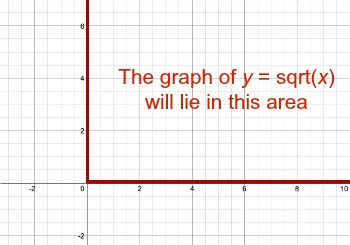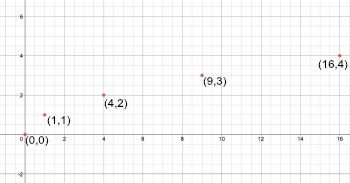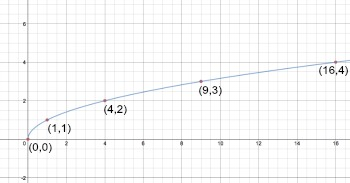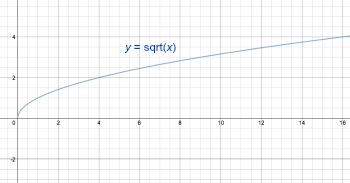# How to Graph y=sqrt(x)

Lesson Transcript
Instructor: Laura Pennington

Laura received her Master's degree in Pure Mathematics from Michigan State University, and her Bachelor's degree in Mathematics from Grand Valley State University. She has 20 years of experience teaching collegiate mathematics at various institutions.

This lesson will show us how to graph the square root function y=sqrt(x). We will look at some of the function's properties, how this graph behaves, and two different ways to go about graphing the function.

## Steps to Solve

In order to graph y = sqrt(x), we first want to get a better idea of how the graph is going to look. We can do this by making some simple observations. The first thing we should take notice of is the domain and range of y = sqrt(x).

The domain consists of all the inputs, or numbers, we plug in for x that make sqrt(x) defined. Since we can't take the square root of negative numbers without getting a non-real number out, and we can't graph non-real numbers on the coordinate axis, the domain of y = sqrt(x) is all real numbers greater than or equal to 0.

The range consists of all of the outputs, or the values y takes on. Based on the fact that our domain consists of all real numbers greater than or equal to 0, the numbers we will get back out will be real numbers greater than or equal to 0. This leads to the following two facts regarding the domain and range of y = sqrt(x):

• The domain of y=sqrt(x) is all real numbers greater than or equal to 0.
• The range of y=sqrt(x) is all real numbers greater than or equal to 0.

This information gives us an idea of where our graph will be on the coordinate axis as is illustrated in this image:Now let's plug in some strategic numbers for x and find the corresponding y values. This will give us an idea of the shape of the graph.

x y
0 0
1 1
4 2
9 3
16 4
25 5

Our chart displays different points that will be on the graph. We can tell that the graph will be rising from left to right since y increases as x increases. We also see that the graph starts off rising fairly quickly, but then rises more and more slowly since the y values go up by 1 as the x values get more and more spaced out.

We now have a general idea of how the graph is behaving. The next thing we need to do is plot some of the points in our chart on a graph.We see the points take on the pattern we expected. The graph will rise from left to right, and it starts off rising quickly, but then slows down. The last thing we need to do is to connect the dots in a smooth line.We've found the following graph to be the graph of y = sqrt(x).An error occurred trying to load this video.

Try refreshing the page, or contact customer support.

Coming up next: Solving the Square Root of i

### You're on a roll. Keep up the good work!

Replay
Your next lesson will play in 10 seconds
• 0:04 Steps to Solve
• 2:23 Graphing Alternative
• 4:07 Lesson Summary
Save Save

Want to watch this again later?

Log in or sign up to add this lesson to a Custom Course.

Timeline
Autoplay
Autoplay
Speed Speed

To unlock this lesson you must be a Study.com Member.
Create your account

### Register to view this lesson

Are you a student or a teacher?

### Unlock Your Education

#### See for yourself why 30 million people use Study.com

##### Become a Study.com member and start learning now.
Back
What teachers are saying about Study.com
Create an account to start this course today
Used by over 30 million students worldwide